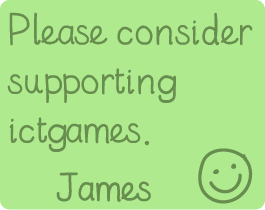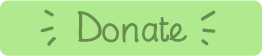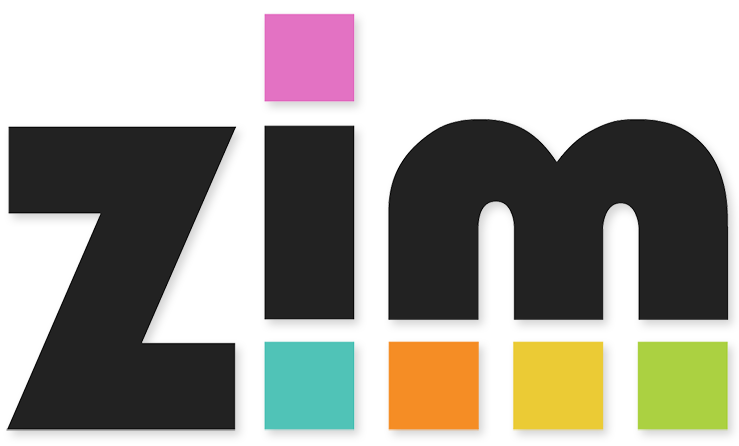# Pop 'n' Drop

### Learning Objectives:

• Y1: To represent and use number bonds and related subtraction facts within 20
• Y1: To add [...] one-digit and two-digit numbers to 20, including zero
• Y2: To recall and use addition [...] facts to 20 fluently, and derive and use related facts up to 100
• Y2: To show that addition of two numbers can be done in any order (commutative) [...]
• Y2: To recall and use multiplication [...] facts for the 2, 5 and 10 multiplication tables [...]
• Y3: To recall and use multiplication and division facts for the 3, 4 and 8 multiplication tables
• Y4: To recall multiplication [...] facts for multiplication tables up to 12 × 12
• Y4: To recognise and write decimal equivalents of any number of tenths or hundredths

### Instructions:

1. Do you want to play an addition or a multiplication game?
1. Drag your finger or mouse over the circles to make your total.
2. You can use as many circles as you need
3. You can go in any direction!
4. You have 2 minutes to find as many as you can.
3. Multiplication game:
1. Drag your finger or mouse over two circles multiply to make your total.
2. You can go in any direction!
3. Some answers will appear on the RHS, choose/press on the correct one.
4. You have 2 minutes to find as many as you can.
This game is ideal for Retrieval Practice. It is low-risk, high reward and high frequency.

Have fun!Made using theandlibraries.